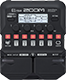# Hi Dist. 1

Discussion in 'Zoom G1/G1X Four' started by Akash Samanta, Nov 6, 2022.

1. hi Dist. 1Device: Zoom G1 Four
Firmware: 2.00

Name on device: Hi Dist. 1
Optimized for: Phones/Speaker

Effects chain:This patch is made only for power cord

Effect: "DIST Plus" (Overdrive / Distortion), active - "yes"
"Gain" = 100
"Volume" = 60
"DryMx" = 0
"Comp" = ORG

Effect: "EP Stomp" (Overdrive / Distortion), active - "yes"
"Gain" = 100
"Bass" = 10
"Treble" = 10
"Volume" = 60

Effect: "HG THRTTL" (Overdrive / Distortion), active - "yes"
"Gain" = 50
"Tone" = 100
"MdCut" = 50
"Volume" = 50

Effect: "Gt GEQ 7" (Filter), active - "yes"
"100" = 12.0
"200" = 12.0
"400" = 12.0
"800" = 0.0
"1.6k" = 12.0
"3.2k" = 12.0
"6.4k" = 12.0
"VOL" = 20

Effect: "ZNR" (Dynamics), active - "yes"
"Detect" = EFXIN
"Depth" = 100
"Threshold" = 100
"Decay" = 0

Patch Volume: 120